# Babuska-Lax-Milgram theorem

(diff) ← Older revision | Latest revision (diff) | Newer revision → (diff)

Many boundary value problems for ordinary and partial differential equations can be posed in the following abstract variational form (cf. also Boundary value problem, ordinary differential equations; Boundary value problem, partial differential equations): Find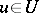such that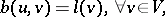(a1)

where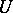andare real normed linear spaces (cf. Norm; Linear space),denotes a functional on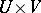andis an element in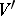(the dual of).

The essential question here is what conditions can be imposed on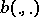and on the normed spacesandso that a unique solution to (a1) exists and depends continuously on the data.

If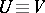is a Hilbert space, P.D. Lax and A.N. Milgram [a1] have proved that for a bilinear continuous functionalstrong coerciveness (i.e., there is asuch that for all,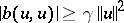) is a sufficient condition for the existence and uniqueness of the solution to (a1) (the Lax–Milgram lemma). In 1971, I. Babuška [a2] gave the following significant generalization of this lemma: Letandbe two real Hilbert spaces and let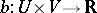be a continuous bilinear functional. If it is also a weakly coercive (i.e., there exists asuch thatandthen for all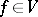there exists a unique solutionsuch that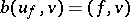for all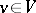and, moreover,.

Sufficient and necessary conditions for a linear variational problem (a1) to have a unique solution depending continuously on the dataare given in [a3], namely: Letbe a Banach space, letbe a reflexive Banach space (cf. Reflexive space) and let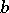be a real functional on. The following statements are equivalent:

i)is a bilinear continuous weakly coercive functional;

ii) there exists a linear, continuous and surjective operator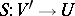such thatfor all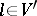and.

This result can be used to give simple examples of bilinear weakly coercive functionals that are not strongly coercive. Indeed, let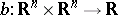be the bilinear functional generated by a square non-singular matrix(i.e.,). Thenis weakly coercive, because for all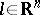there exists a unique solution,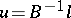, for (a1); however, it is strongly coercive if and only ifis either strictly positive (i.e.,for all) or strictly negative (i.e.,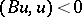for all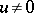).

Using this fact one can prove that if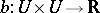is symmetric (i.e.,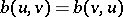) and strictly defined (i.e.,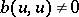for all), then it is either a strictly positive functional (i.e.,for all) or a strictly negative functional (i.e.,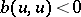for all); moreoverfor all. The following result can also be found in [a3]: Ifis a symmetric and continuous functional then it is strongly coercive if and only if it is weakly coercive and strictly defined. This implies that ifis a symmetric and strictly defined functional, then it is strongly coercive if and only if it is weakly coercive.

Effective applications of the Babuška–Lax–Millgram theorem can be found in [a4].